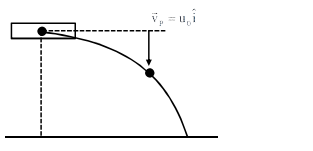# A bomb is dropped by fighter plane flying horizontally. To an observer sitting in the plane, the trajectory of the bomb is a :`
Question:

A bomb is dropped by fighter plane flying horizontally. To an observer sitting in the plane, the trajectory of the bomb is a :

1. hyperbola

2. parabola in the direction of motion of plane

3. straight line vertically down the plane

4. parabola in a direction opposite to the motion of plane

Correct Option: , 3

Solution:$\mathrm{v}_{\mathrm{B}}=\mathrm{u}_{0} \hat{\mathrm{i}}-\mathrm{gt} \hat{\mathrm{j}}$

$\overrightarrow{\mathrm{v}}_{\mathrm{B} / \mathrm{P}}=\overrightarrow{\mathrm{v}}_{\mathrm{B}}-\overrightarrow{\mathrm{v}}_{\mathrm{P}}$

$\overrightarrow{\mathrm{v}}_{\mathrm{B} / \mathrm{P}}=-8 \mathrm{t} \mathbf{j}$

straight line vertically down

Ans. 3